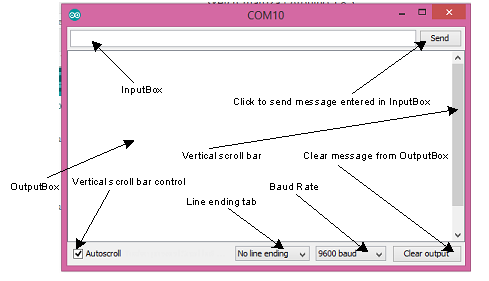# How to use Serial.parseInt

when I input

16 17 18 19 20 21 22 23 24 25

there arent react.why?

``````int buf;
int inChar;
boolean stringComplete = false;
int i=0,k,h;

void setup()
{
Serial.begin(115200);
}

void loop() {
while(Serial.available()>0)
{
inChar = Serial.parseInt();

if(inChar == '\n') {
stringComplete = true;
}
else if(i<500){
buf[i++] = inChar;
}
}

if(stringComplete)
{
stringComplete = false;
for(k=0;k<30;k++){
Serial.print("=");
}
Serial.println();
Serial.print("Length of input data : ");
Serial.print(i);
Serial.println(" integers");
for(k=0;k<i;k++){
Serial.print(buf[k]);
Serial.print(" ");
}
Serial.println();
i = 0;
h = 0;
}
}
``````
``````    inChar = Serial.parseInt();

if(inChar == '\n') {
stringComplete = true;
``````

You are reading in a number (skipping any spaces before it) and then checking to see if the number is '\n'. A newline character is 10 (0x0A)and your numbers start at 16 and go up from there. Try typing " 10 " at the end of the line.

A read of Robin's Serial Input Basics - updated might give you some ideas how to implement serial communication.